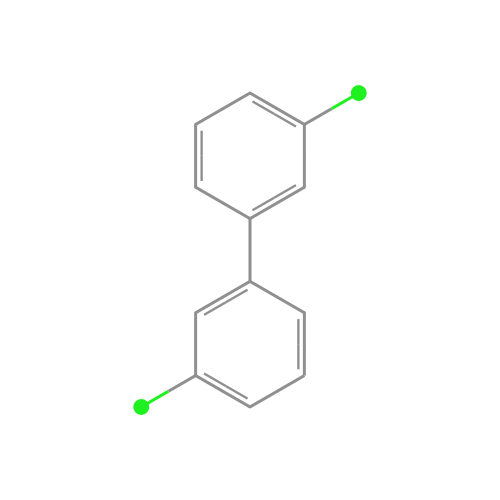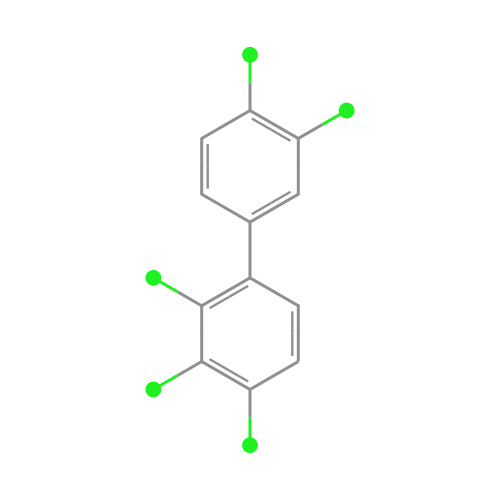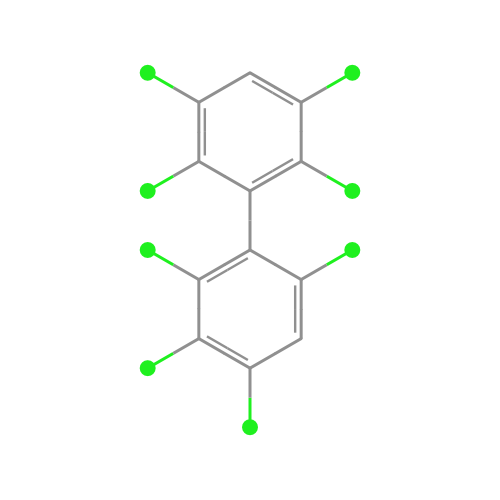## Developer Blog# Bayesian Binomial Test in R

### exact estimation of a Bayesian binomial test, with a baseball example

Summary: in this post, I implemenent an R function for computing $$P(\theta_1 > \theta2)$$, where $$\theta_1$$ and $$\theta_2$$ are beta-distributed random variables. This is useful for estimating the probability that one binomial proportion is greater than another.

I am working on a project in which I need to compare two binomial proportions to see if one is likely to be greater than the other. I wanted to do this in a Bayesian framework; fortunately, estimating binomial proportions is one of the first subjects taught to introduce Bayesian statistics (and statistics in general, for that matter) so the method is well established. Rasmus Bååth has a very nice article describing the Bayesian binomial test, and an estimation approach using JAGS.

I made the problem even simpler by using the fact that the beta distribution is the conjugate prior to the binomial distribution. That is, if the prior is $$\mathrm{beta}(\alpha, \beta)$$ distributed, then the posterior after observing $$k$$ successes in $$n$$ trials is

If you want to start with a noninformative prior, then you can use the beta distribution with $$\alpha = \beta = 1$$, which collapses to the uniform distribution. This makes the posterior

What I am interested is in comparing two binomial proportions, $$\theta_1$$ and $$\theta_2$$. I want to calculate

We observe $$k_1$$ successes out of $$n_1$$ trials and estimate a posterior distribution for $$\theta_1$$, and $$k_2$$ successes out of $$n_2$$ trials for $$\theta_2$$. Then the probability in question is

So far, so textbook. At this point, the simplest way to get an estimate is to draw two sets of random numbers, distributed by the two respective beta distributions, and see how many times the first random numbers is greater than the second:

This works fine, but it would be nice to have an exact solution. I found several papers describing different approaches.

# A Bayesian approach to modelling censored data

### fitting models to censored data with STAN

For thise case, we can write Bayes formula as:

The two components in the numerator are:

• The probability of the data given a $$\mu$$ and $$\sigma$$, also called the likelihood function: $$p(y|\mu,\sigma)$$
• The probability of a given $$\mu$$ and $$\sigma$$, before seeing any data; also called the prior likelihood: $$p(\mu, \sigma)$$

The denominator, $$p(y^{\ast})$$, is the total likelihood of the data integrated over all hypotheses (that is, over all values of $$\mu$$ and $$\sigma$$). This is the part of the formula that typically doesn’t have an analytical solution; in practice, numerical approximations need to be used.

Our job is to define the likelihood function and provide a prior distribution for $$\mu$$ and $$\sigma$$.

In the previous post, we derived the likelihood function for left-censored data:

where $$P$$ is the log-normal probability density function and $$D$$ is the log-normal cumulative density function.

The specification of a prior distribution depends on the application, and what is known about what reasonable values may be for $$\mu$$ and $$\sigma$$. In this case, let’s set a very weakly informative prior on both variables: $$\mu \sim Normal(0, 100)$$ and $$\sigma \sim Normal(0, 100)$$

A STAN program that encodes our likelihood function and prior specifications:

This program is written to look like the math, so it doesn’t use some special syntax that makes the code shorter, and it isn’t as computationally efficient as it code be.

data {
int<lower=0> N;
real L; // censoring limit
real<lower=L> y[N];
int cens[N]; // -1 if left-censored, 0 if not censored
}
parameters {
real mu;
real<lower=0> sigma;
}
model {
// priors
mu ~ normal(0, 100);
sigma ~ normal(0, 100);

// likelihood
for(i in 1:N) {
if(cens[i] == -1) {
// left-censored
target += normal_cdf(L | mu, sigma);
}
else {
// not censored
target += normal_pdf(y[i] | mu, sigma);
}
}
}


# Unlocking Data in PDFs

### our workflow for scraping data

Unfortunately, there is a lot of data released in on the web in the form of PDF files. Scraping data out of PFDs is much harder than scraping from a web page; web pages have structure, in the form of HTML, that you can usually leverage to extract structured data.

It isn’t hopeless, it’s just harder. Here are some of the tools and techniques that we’ve found useful in parsing data from PDFs.

# pdftotext

pdftotext is a utility from the Xpdf project that converts PDFs to flat text files. It is easiest to install and use on unix based platforms, where it can be found in the poppler-utils package. There is also a windows port of Xpdf that I’ve used successfully.

pdftotext has several useful flags that effect how it parses its input:

• -layout
• -raw

# tabula

Recently I’ve switched to Tabula for most of my PDF scraping needs. Tabula is a desktop application for extracting data from PDFs. I’ve found it to be more reliable than pdftotext. The only drawback is that it isn’t a command line program, so automating the scraping isn’t as easy as pdftotext. On the other hand, you can visually select the parts of the PDFs you’d like to scrape, which is useful for one-off jobs.

# Tracking Packages in Excel

### using VBA to track UPS packages

We’ve been shipping hundreds of packages as part of our Detox Me Action Kit project, and we wanted a way to track all of them without having to manually enter their tracking numbers on the UPS website. Through the UPS REST API and some VBA hacking, we were able to retrieve tracking information directly from Excel.

# NHANES made simple with RNHANES

### spend less time munging, and more time analyzing

Scientists spend a lot of time “munging” data. Finding, cleaning, and managing datasets can take up the majority of the time it takes to complete an analysis. Tools that make the munging process easier can save scientists a lot of time.

We are tackling a small part of this problem in the context of the CDC’s National Health and Nutrition Examination Survey, a national study conducted every two years that gathers data on American’s health, nutrition, and exposure to environmental chemicals. Data from NHANES is used widely by environmental health scientists to study the U.S. public’s exposure to chemicals.

Figuring out how to set up a proper analysis of NHANES data can be tricky if you’re starting from scratch. That’s why we wrote RNHANES, an R package that makes it easy to download, analyze, and visualize NHANES data. RNHANES streamlines accessing the data, helps to set up analyses that correctly incorporate the study’s sample weights, and has built in plotting functions.

Let’s take the package for a spin by conducting a real-world analysis of NHANES data. Specifically, let’s characterize the U.S. population’s exposure to PFOA, a perfluo-alkyl substance (PFAS, also called “highly fluorinated compound”) that is linked to a number of adverse health effects.# Fitting censored log-normal data

### exploration of maximum-likelihood estimation techniques

Data are censored when samples from one or both ends of a continuous distribution are cut off and assigned one value. Environmental chemical exposure datasets are often left-censored: instruments can detect levels of chemicals down to a limit, underneath which you can’t say for sure how much of the chemical was present. The chemical may not have been present at all, or was present at a low enough level that the instrument couldn’t pick it up.

There are a number of methods of dealing with censored data in statistics. The crudest approach is to substitute a value for every censored value. This may be zero, the detection limit, the detection limit over the square root of two, or some other value. This method, however, biases the statistical results depending on what you choose to substitute.

A more nuanced alternative is to assume the data follows a particular distribution. In the case of environmental data, it often makes sense to assume a log-normal distribution. When we do this, while we may not know what the precise levels of every non-detect, we can at least assume that they follow a log-normal distribution.

In this article, we will walk through fitting a log-normal distribution to a censored dataset using the method of maximum likelihood.# ggplot2 axis limit gotchas

### axis limits remove data by default

Setting axis limits in ggplot has behaviour that may be unexpected: any data that falls outside of the limits is ignored, instead of just being hidden. This means that if you apply a statistic or calculation on the data, like plotting a box and whiskers plot, the result will only be based on the data within the limits.

In other words, ggplot doesn’t “zoom in” on a part of your plot when you apply an axis limit, it recalculates a new plot with the restricted data.We wanted a way to generate beautiful chemical structure visualizations, like the ones you see above, for use in our materials. We developed a lightweight tool called sdftosvg that does just that: it takes as input SDF files, downloadable from repositories like PubChem, and outputs SVG renderings.